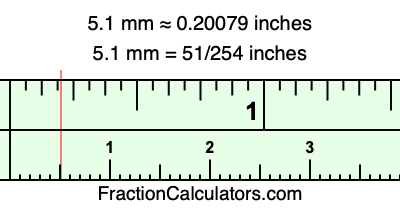5.1 mm in inchesHere is how to convert 5.1 mm to inches. We will give you the fractional answer, the decimal answer, and illustrate the answer on our tape measure.

One millimeter is equal to 5/127 inches, and 5.1 as a fraction is 51/10. Therefore, to get the fractional answer to 5.1 mm in inches, we multiply 51/10 by 5/127 and simplify if necessary.

cm × 5/127 = inches
51/10 × 5/127 = inches
51/10 × 5/127 = 51/254
51/10 mm = 51/254 inches
5.1 mm = 51/254 inches

As promised above, we also have the answer to 5.1 mm in inches in decimal form. Although the fractional answer above is exact, the decimal answer may be rounded if necessary:

51/254 ≈ 0.200787401574803
51/254 ≈ 0.20079
5.1 mm ≈ 0.20079 inches

Our image below shows 5.1 mm on a tape measure. The top row of the tape measure is inches, and each mark on the bottom row is 1 millimeter (10 millimeter marks equal 1 centimeter).As you can see, we drew a red line where 5.1 mm and 0.20079 inches meet on the measuring tape.

Millimeters to Inches Calculator
Here you can convert another length in millimeters to inches.

Convert  mm to inches.

What is 5.2 mm in inches?
Here is another inches to millimeters calculation that we have done for you.